Important Coordinate Geometry Questions

Chapter 7 Class 10 Coordinate Geometry
Serial order wise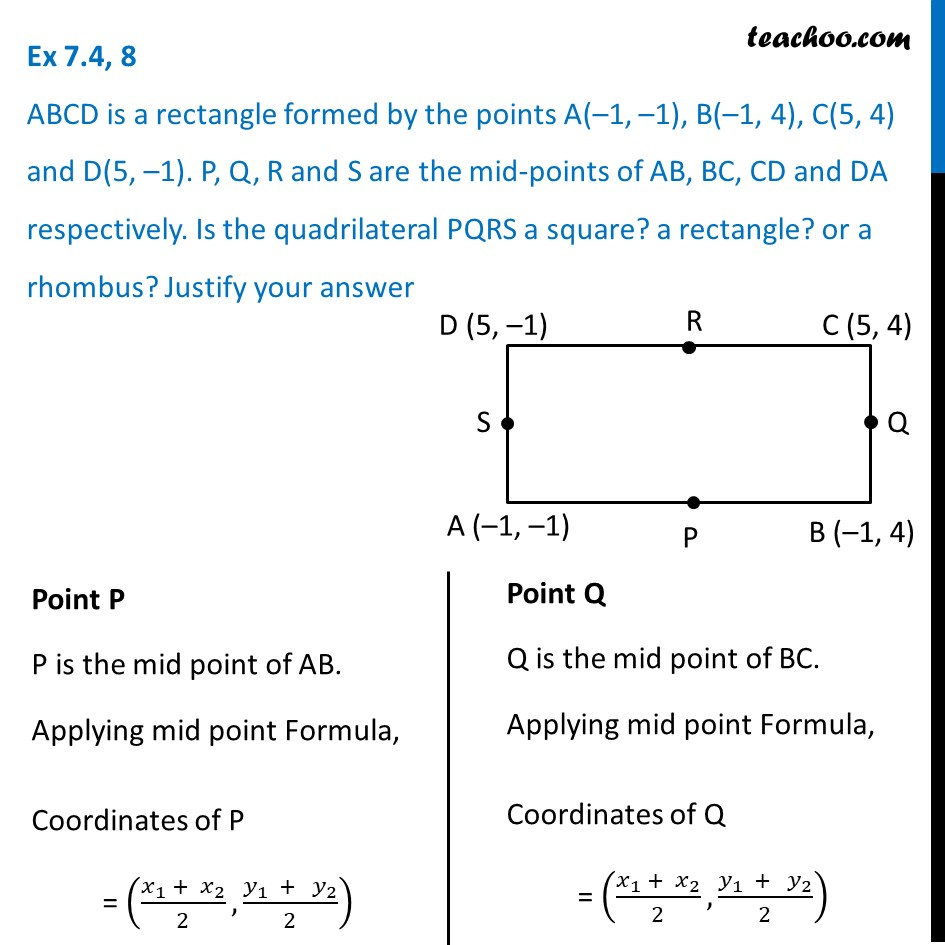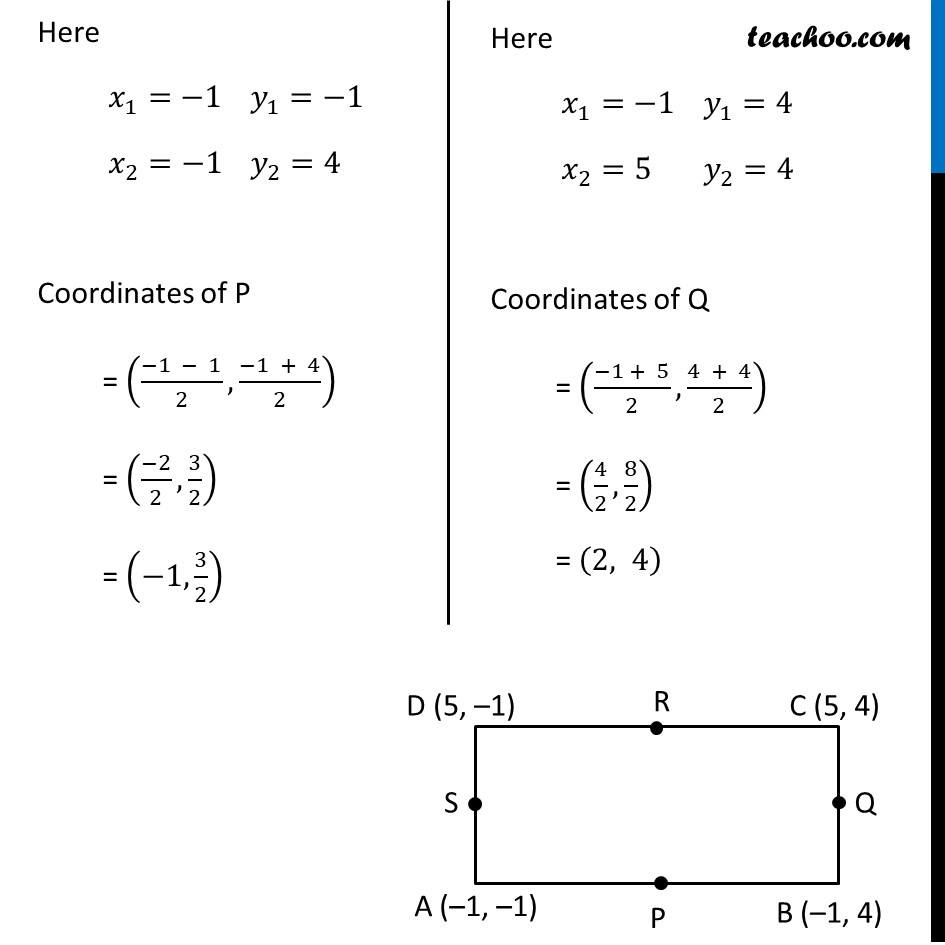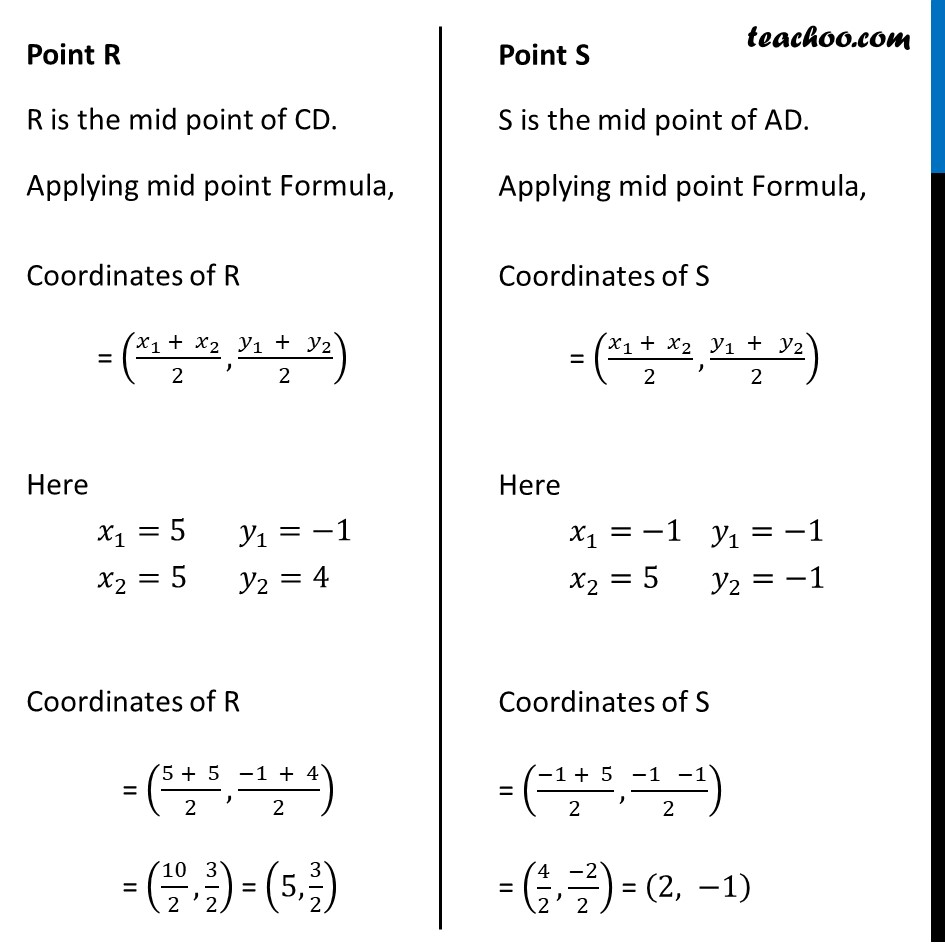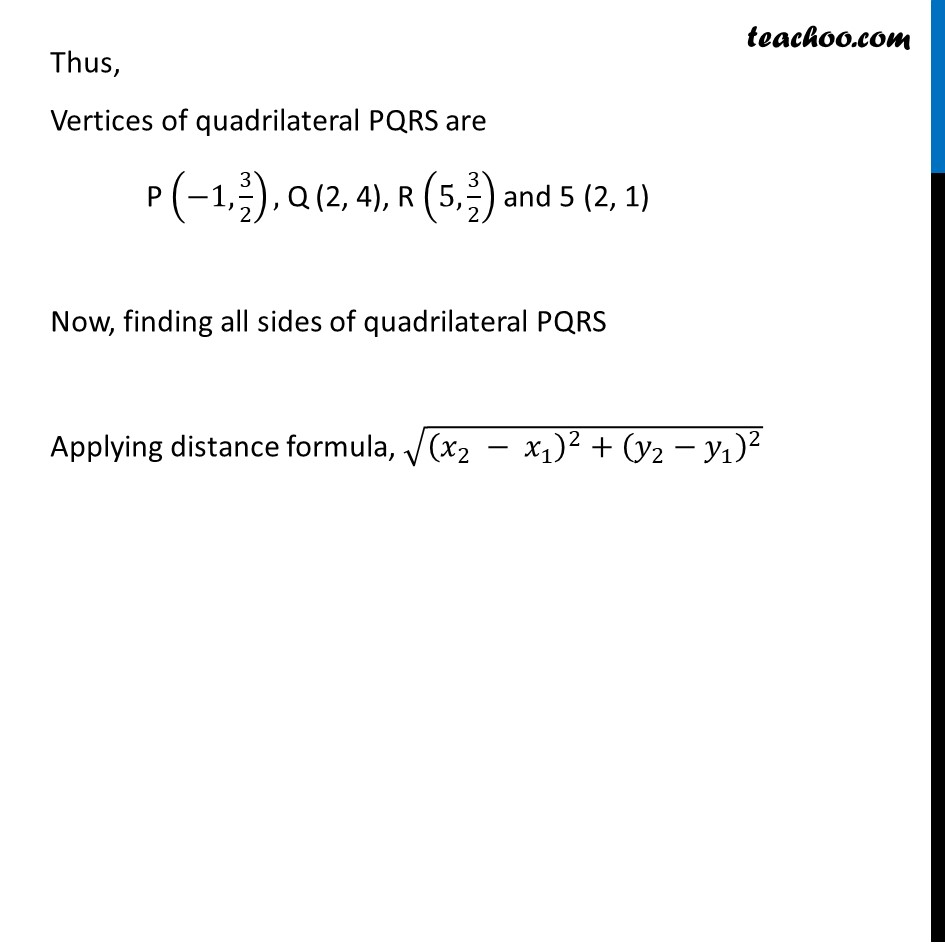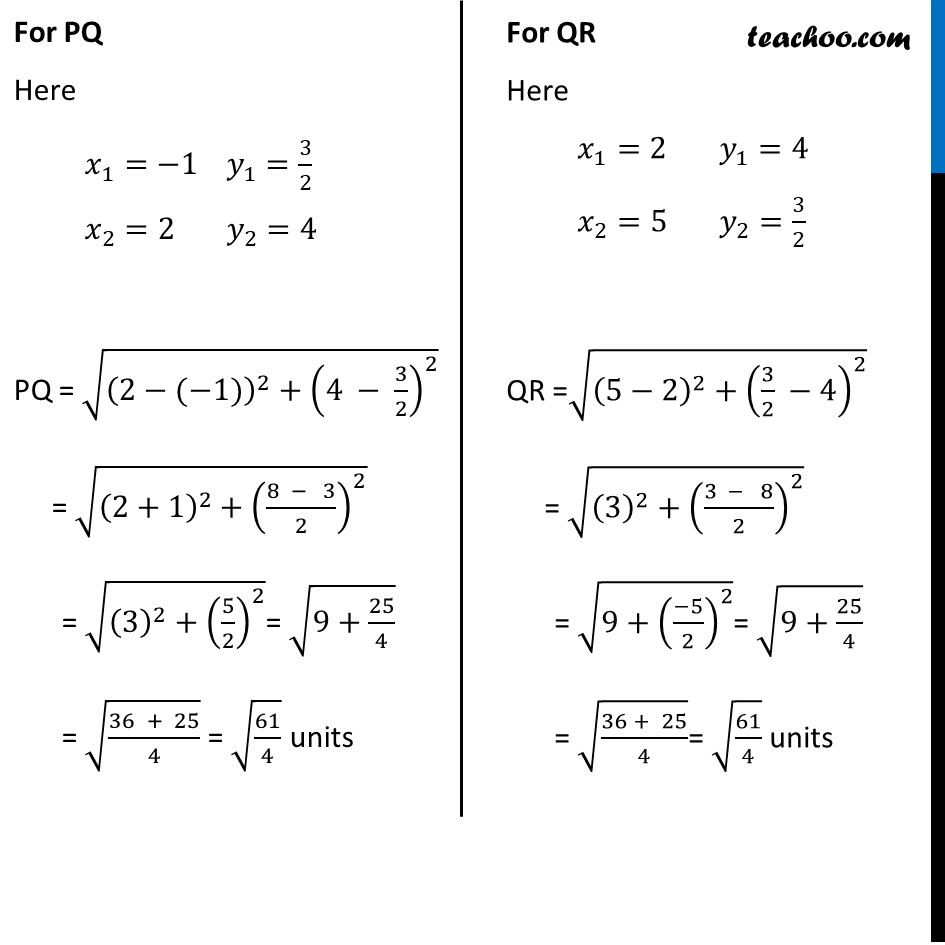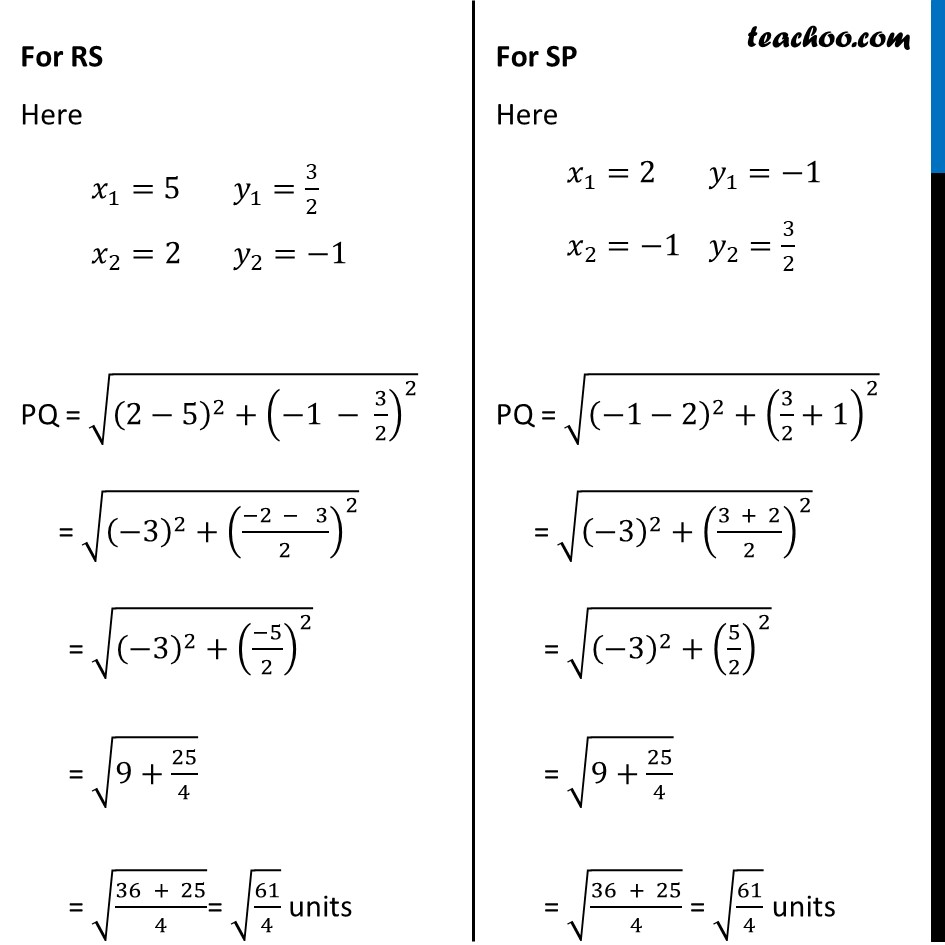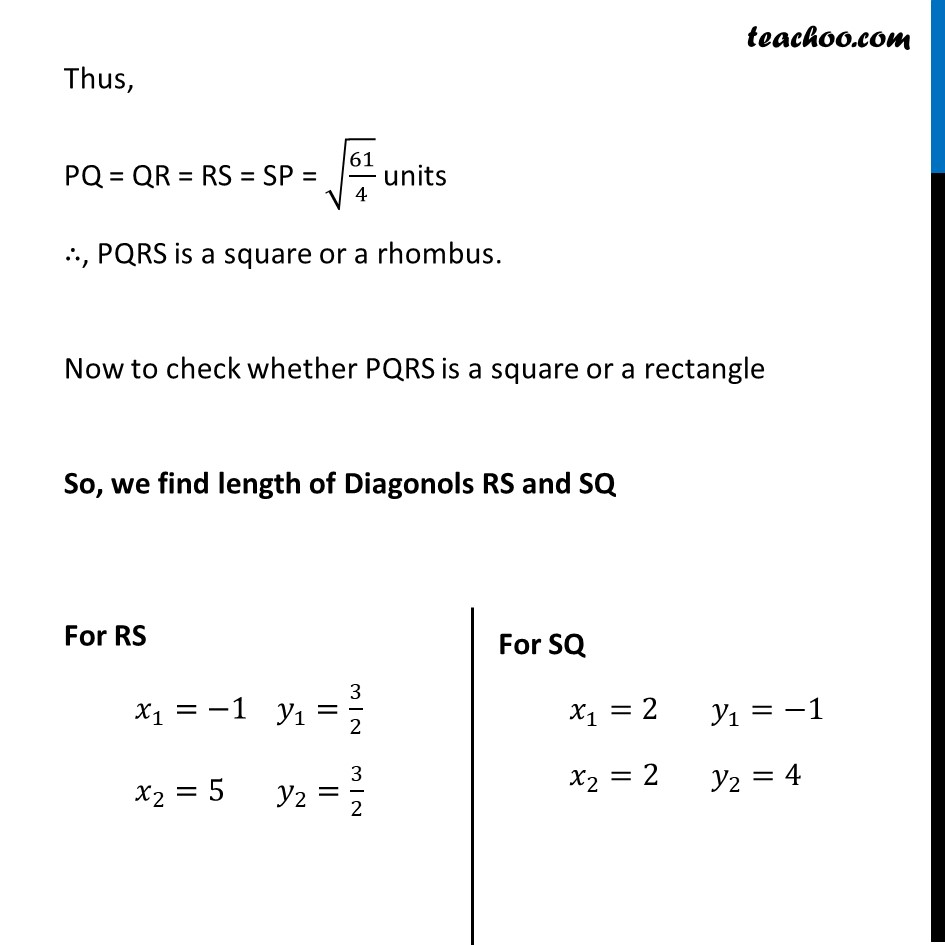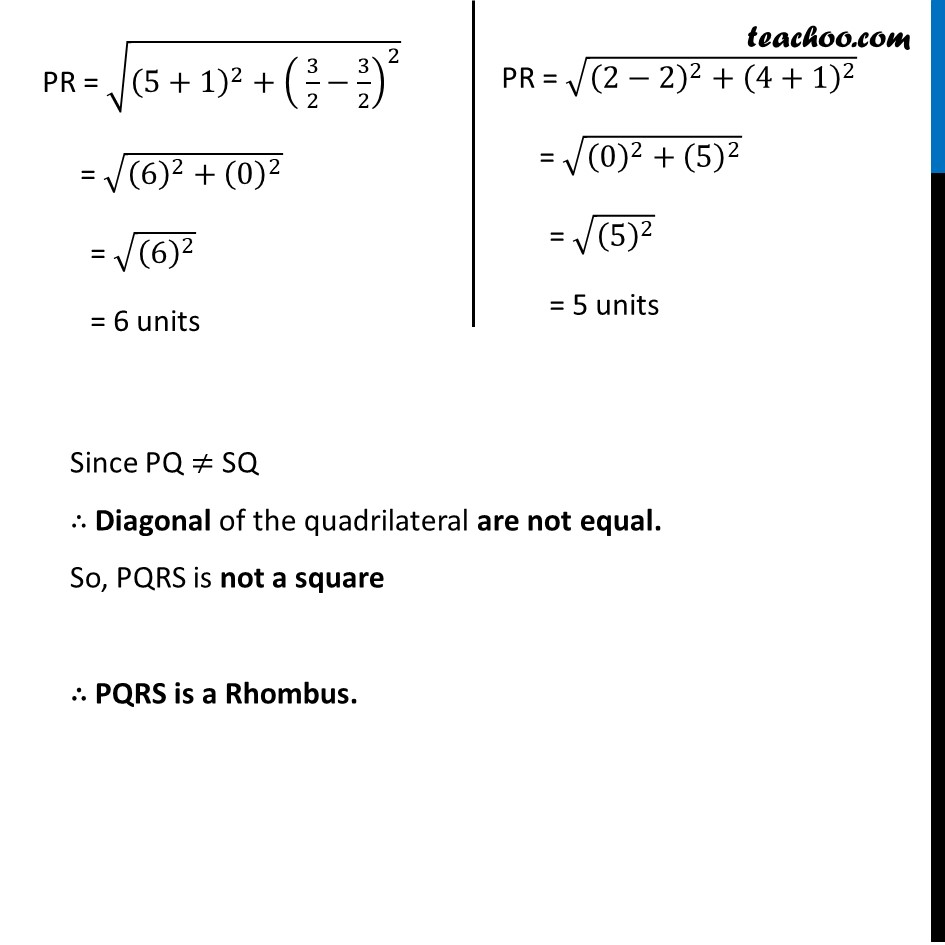Learn in your speed, with individual attention - Teachoo Maths 1-on-1 Class

### Transcript

Question 8 ABCD is a rectangle formed by the points A(–1, –1), B(–1, 4), C(5, 4) and D(5, –1). P, Q, R and S are the mid-points of AB, BC, CD and DA respectively. Is the quadrilateral PQRS a square? a rectangle? or a rhombus? Justify your answer Point P P is the mid point of AB. Applying mid point Formula, Coordinates of P = ((𝑥_1 +〖 𝑥〗_2)/2, (𝑦_1 + 𝑦_2)/2) Point Q Q is the mid point of BC. Applying mid point Formula, Coordinates of Q = ((𝑥_1 +〖 𝑥〗_2)/2, (𝑦_1 + 𝑦_2)/2) Here 𝑥_1=−1 𝑦_1=−1 𝑥_2=−1 𝑦_2=4 Coordinates of P = ((−1 − 1)/2, (−1 + 4)/2) = ((−2)/2,3/2) = (−1, 3/2) Here 𝑥_1=−1 𝑦_1=4 𝑥_2=5 𝑦_2=4 Coordinates of Q = ((−1 + 5)/2, (4 + 4)/2) = (4/2,8/2) = (2, 4) Point R R is the mid point of CD. Applying mid point Formula, Coordinates of R = ((𝑥_1 +〖 𝑥〗_2)/2, (𝑦_1 + 𝑦_2)/2) Here 𝑥_1=5 𝑦_1=−1 𝑥_2=5 𝑦_2=4 Coordinates of R = ((5 + 5)/2, (−1 + 4)/2) = (10/2,3/2) = (5, 3/2) Point S S is the mid point of AD. Applying mid point Formula, Coordinates of S = ((𝑥_1 +〖 𝑥〗_2)/2, (𝑦_1 + 𝑦_2)/2) Here 𝑥_1=−1 𝑦_1=−1 𝑥_2=5 𝑦_2=−1 Coordinates of S = ((−1 + 5)/2, (−1 −1)/2) = (4/2,(−2)/2) = (2, −1) Thus, Vertices of quadrilateral PQRS are P (−1, 3/2), Q (2, 4), R (5, 3/2) and 5 (2, 1) Now, finding all sides of quadrilateral PQRS Applying distance formula, √((𝑥_2 − 𝑥_1 )^2+(𝑦_2−𝑦_1 )^2 ) For PQ Here 𝑥_1=−1 𝑦_1=3/2 𝑥_2=2 𝑦_2=4 PQ = √((2−(−1))^2+(4 − 3/2)^2 ) = √((2+1)^2+((8 − 3)/2)^2 ) = √((3)^2+(5/2)^2 )= √(9+25/4) = √((36 + 25)/4) = √(61/4) units For QR Here 𝑥_1=2 𝑦_1=4 𝑥_2=5 𝑦_2=3/2 QR =√((5−2)^2+(3/2 −4)^2 ) = √((3)^2+((3 − 8)/2)^2 ) = √(9+((−5)/2)^2 )= √(9+25/4) = √((36 + 25)/4)= √(61/4) units For RS Here 𝑥_1=5 𝑦_1=3/2 𝑥_2=2 𝑦_2=−1 PQ = √((2−5)^2+(−1 − 3/2)^2 ) = √((−3)^2+((−2 − 3)/2)^2 ) = √((−3)^2+((−5)/2)^2 ) = √(9+25/4) = √((36 + 25)/4)= √(61/4) units For SP Here 𝑥_1=2 𝑦_1=−1 𝑥_2=−1 𝑦_2=3/2 PQ = √((−1−2)^2+(3/2+1)^2 ) = √((−3)^2+((3 + 2)/2)^2 ) = √((−3)^2+(5/2)^2 ) = √(9+25/4) = √((36 + 25)/4) = √(61/4) units Thus, PQ = QR = RS = SP = √(61/4) units ∴, PQRS is a square or a rhombus. Now to check whether PQRS is a square or a rectangle So, we find length of Diagonols RS and SQ For RS 𝑥_1=−1 𝑦_1=3/2 𝑥_2=5 𝑦_2=3/2 For SQ 𝑥_1=2 𝑦_1=−1 𝑥_2=2 𝑦_2=4 PR = √((5+1)^2+( 3/2−3/2)^2 ) = √((6)^2+(0)^2 ) = √((6)^2 ) = 6 units PR = √((2−2)^2+(4+1)^2 ) = √((0)^2+(5)^2 ) = √((5)^2 ) = 5 units Since PQ ≠ SQ ∴ Diagonal of the quadrilateral are not equal. So, PQRS is not a square ∴ PQRS is a Rhombus.